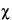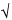Search USP29

GLOSSARY
Glossary of Symbols
 A absorbance for computing % reduction in bacte-rial growth from turbidimetric readings. b slope of the straight line relating response (y) tolog-dose (x) [Equations 2b, 4, 5, 6]. c constant for computing M¢ with Equations 8and 10. c¢ constant for computing L with Equations 26and 29. ci constant for computing M¢ when doses are spacedas in Table 8. c¢i2 constant for computing L when doses are spacedas in Table 8. C term measuring precision of the slope in aconfidence interval [Equations 27, 28, 35, 36].2 statistical constant for testing significance of adiscrepancy [Table 9].M22 testing the disagreement between differentestimates of log-potency [Equations 39, 40]. eb ei from row b in Tables 6 to 8. eb¢i multiple of S(x – bar(x))2 [Table 5; Equation 6]. ei sum of squares of the factorial coefficients in eachrow of Tables 6 to 8. eq ei from row q in Tables 6 to 8. f number of responses at each dosage level of apreparation; number of replicates or sets. fS number of observations on the Standard. fU number of observations on the Unknown. F1 to F3 observed variance ratio with 1 to 3 degrees offreedom in numerator [Table 9]. G1, G2, and G3 relative gap in test for outlier [Table 1]. h number of Unknowns in a multiple assay. h¢ number of preparations in a multiple assay,including the Standard and h Unknowns; i.e.,h¢ = h + 1. i interval in logarithms between successivelog-doses, the same for both Standardand Unknown. k number of estimated log-potencies in an average[Equation 24]; number of treatments or doses[Table 4; Equations 1, 13, 15, 16]; number ofranges or groups in a series [Table 2]; numberof rows, columns, and doses in a single Latinsquare [Equations 1a, 16a]. L length of the confidence interval in logarithms[Equations 24, 26, 29, 38], or in terms of aproportion of the relative potency of thedilutions compared [Equations 31, 33]. Lc length of a combined confidence interval[Equations 42, 43]. Lc¢ length of confidence interval for a semi-weightedmean bar(M) [Equation 48]. LD50 lethal dose killing an expected 50% of theanimals under test [Equation 2c]. M log-potency [Equation 2]. M¢ log-potency of an Unknown, relative to itsassumed potency. bar(M) mean log-potency. n degrees of freedom in an estimated variance s2 orin the statistic t or2. n¢ number of Latin squares with rows in common[Equations 1a, 16a]. N number; e.g., of observations in a gap test[Table 1], or of responses y in an assay[Equation 16]. P probability of observing a given result, or of thetabular value of a statistic, usually P = 0.05 or0.95 for confidence intervals [ Tables 1, 2, 9]. P* potency, P* = antilog M or computed directly. R ratio of a given dose of the Standard to thecorresponding dose of the Unknown, or assumed potencyof the Unknown [Equations 2, 30, 33]. R* ratio of largest of k ranges in a series to their sum[Table 2]. s =bar(s)2 standard deviation of a response unit, also of asingle estimated log-potency in a direct assay[Equation 24]. s2 error variance of a response unit. Si a log-dose of Standard [ Tables 6, 7]. S “the sum of.” t Student's t for n degrees of freedom andprobability P = 0.05 [Table 9]. T total of the responses y in an assay [Equation 16]. T ¢ incomplete total for an assay in randomized setswith one missing observation [Equation 1]. T1 S(y) for the animals injected with the Standard onthe first day [Equations 18, 36]. T2 S(y) for the animals injected with the Standard onthe second day [Equations 18, 36]. Ta Ti for the difference in the responses to theStandard and to the Unknown [Tables 6 to 8]. Tab Ti for testing the difference in slope betweenStandard and Unknown [Tables 6 to 8]. Taq Ti for testing opposed curvature in the curves forStandard and Unknown [Tables 6 to 8]. Tb Ti for the combined slope of the dosage-responsecurves for Standard and Unknown [Tables 6 to8]. Tb¢ S(x1Tt) or S(x1y) for computing the slope of thelog-dose response curve [Equations 10, 23,28]. Ti sum of products of Tt multiplied by thecorresponding factorial coefficients in eachrow of Tables 6 to 8. Tq Ti for testing similar curvature in the curves forStandard and Unknown [Tables 6 to 8]. Tr row or set total in an assay in randomized sets[Equation 16]. Tr¢ incomplete total for the randomized set with amissing observation in Equation 1. Tt total of f responses y for a given dose of apreparation [Tables 6 to 8; Equations 6, 13,14, 16]. Tt¢ incomplete total for the treatment with a missingobservation in Equation 1. Ui a log-dose of Unknown [Tables 6 to 8]. v variance for heterogeneity betweenassays [Equation 45]. V = 1/w variance of an individual M [Equations 44 to 47]. w weight assigned to the M for an individual assay[Equation 38], or to a probit for computing anLD50 [Equations 2a, 2b]. w¢ semi-weight of each M in a series of assays[Equations 47, 48]. x a log-dose of drug in a bioassay [Equation 5];also the difference between two log-thresholddoses in the same animal [Equation 12]. x* coefficients for computing the lowest and highestexpected responses YL and YH in a log-doseresponse curve [Table 4; Equation 3]. x1 a factorial coefficient that is a multiple of (x – bar(x))for computing the slope of a straight line[Table 5; Equation 6]. bar(x) mean log-dose [Equation 5]. bar(x)S mean log-dose for Standard [Equation 9]. bar(x)U mean log-dose for Unknown [Equation 9]. X log-potency from a unit response, as interpolatedfrom a standard curve [Equations 7a, 7b, 19]. XM confidence limits for an estimated log-potency M[Equations 25, 30]. XP* confidence limits for a directly estimatedpotency P* (see Digitalis assay) [Equation 33]. y an observed individual response to a dose of drugin the units used in computing potency and theerror variance [Equations 13 to 16]; a unitdifference between paired responses in 2-doseassays [Equations 17, 18]. y1 . . . yN observed responses listed in order of magnitude,for computing G1, G2, or G3 in Table 1. y¢ replacement for a missing value [Equation 1]. bar(y) mean response in a set or assay [Equation 5]. bar(y)t mean response to a given treatment[Equations 3, 6]. Y a response predicted from a dosage-responserelationship,often with qualifying subscripts[Equations 3 to 5]. z threshold dose determined directly by titration(see Digitalis assay) [Equation 11]. bar(z) mean threshold dose in a set (see Digitalis assay)[Equations 31, 32, 33].